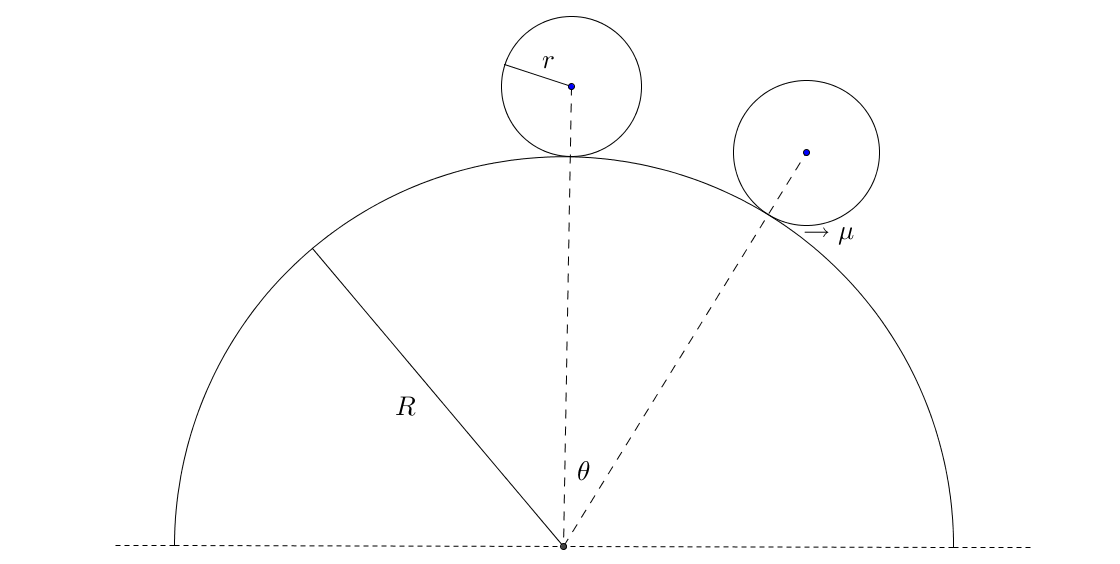# Ball, Don't Fall! - Part 2A solid spherical ball of radius $r=2 \ \text{m}$ is carefully placed on top of a fixed hemisphere of radius $R=10 \ \text{m}$ as shown. It is then pushed very slightly.

If the ball doesn't slip till it makes an angle $\theta=30^{\text{o}}$ with the vertical, then the minimum required value of coefficient of friction between the ball and the hemisphere is $\dfrac{2}{a\sqrt{b}-c}$

where $a,b$ and $c$ are co-prime positive integers. Find $a+b+c$

Details And Assumptions

• Take $g=9.8 \ \text{m}\text{s}^{-2}$ in the downward direction if needed.
×